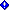The XmlReader sample demonstrates the processing of a message body using an XmlReader. The sample is based on the Getting Started Sample, which implements a calculator service. An additional service operation, `Sum`, has been added that accepts a message that contains an array of values to add together. The service reads the message using an XmlReader.

Note

The setup procedure and build instructions for this sample are located at the end of this topic.

The calculator interface includes a service operation named `Sum` that accepts a Message parameter, as shown in the following sample code.

``````public interface ICalculator
{
[OperationContract]
[OperationContract]
double Subtract(double n1, double n2);
[OperationContract]
double Multiply(double n1, double n2);
[OperationContract]
double Divide(double n1, double n2);
[OperationContract]
Message Sum(Message message);
}
``````

The client accesses `Sum` by first creating an array of integer values, then creating a message from the array, and then calling the `Sum` method using the created message, as shown in the following sample code.

``````CalculatorClient client = new CalculatorClient();
...
// Call the Sum service operation.
int[] values = { 1, 2, 3, 4, 5 };
using (new OperationContextScope(client.InnerChannel))
{
Message request = Message.CreateMessage(OperationContext.Current.OutgoingMessageHeaders.MessageVersion, "http://Microsoft.ServiceModel.Samples/ICalculator/Sum", values);

Console.WriteLine("Sum(1,2,3,4,5) = {0}", sum);
}
``````

In the service, the implementation of the service operation `Sum` accesses the message body using an XmlReader object to iterate through the values to sum. The GetReaderAtBodyContents method is called to access the message body, as shown in the following sample code.

``````public int Sum(Message message)
{
int sum = 0;
string text = "";

//The body of the message contains a list of numbers that are read
{
if (text.Length>0)
{
sum += Convert.ToInt32(text);
}
}
body.Close();
Message response = Message.CreateMessage(
"http://Microsoft.ServiceModel.Samples/ICalculator/SumResponse",
sum);
return response;
}
``````

When you run the sample, the requests and responses of the operation are displayed in the client console window. Press ENTER in the client window to shut down the client.

``````Add(100,15.99) = 115.99
Subtract(145,76.54) = 68.46
Multiply(9,81.25) = 731.25
Divide(22,7) = 3.14285714285714
Sum(1,2,3,4,5) = 15

Press <ENTER> to terminate client.
``````

#### To set up, build, and run the sample

1. Ensure that you have performed the One-Time Setup Procedure for the Windows Communication Foundation Samples.

2. To build the C# or Visual Basic .NET edition of the solution, follow the instructions in Building the Windows Communication Foundation Samples.

3. To run the sample in a single- or cross-machine configuration, follow the instructions in Running the Windows Communication Foundation Samples.Note:
The samples may already be installed on your machine. Check for the following (default) directory before continuing.

`<InstallDrive>:\WF_WCF_Samples`

If this directory does not exist, go to Windows Communication Foundation (WCF) and Windows Workflow Foundation (WF) Samples for .NET Framework 4 to download all Windows Communication Foundation (WCF) and WF samples. This sample is located in the following directory.

`<InstallDrive>:\WF_WCF_Samples\WCF\Basic\Contract\Message\XmlReader`